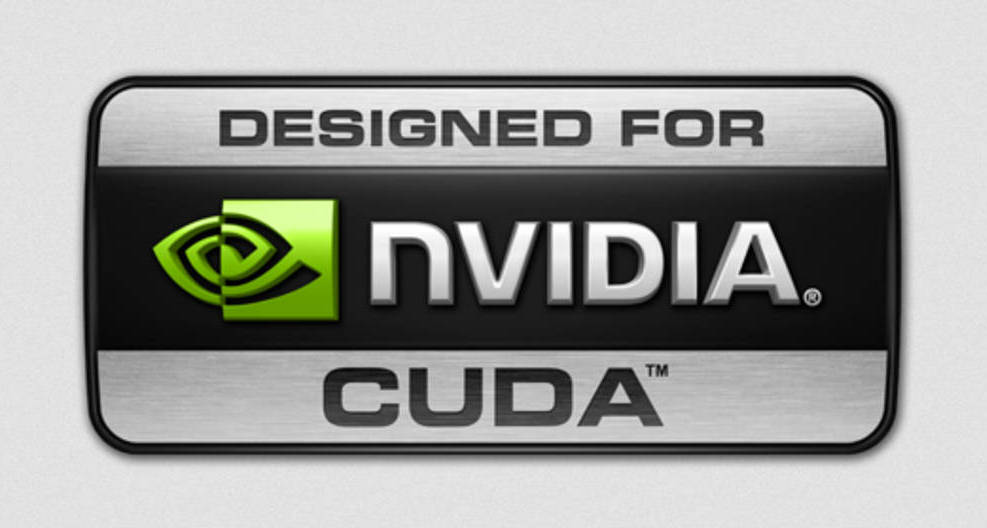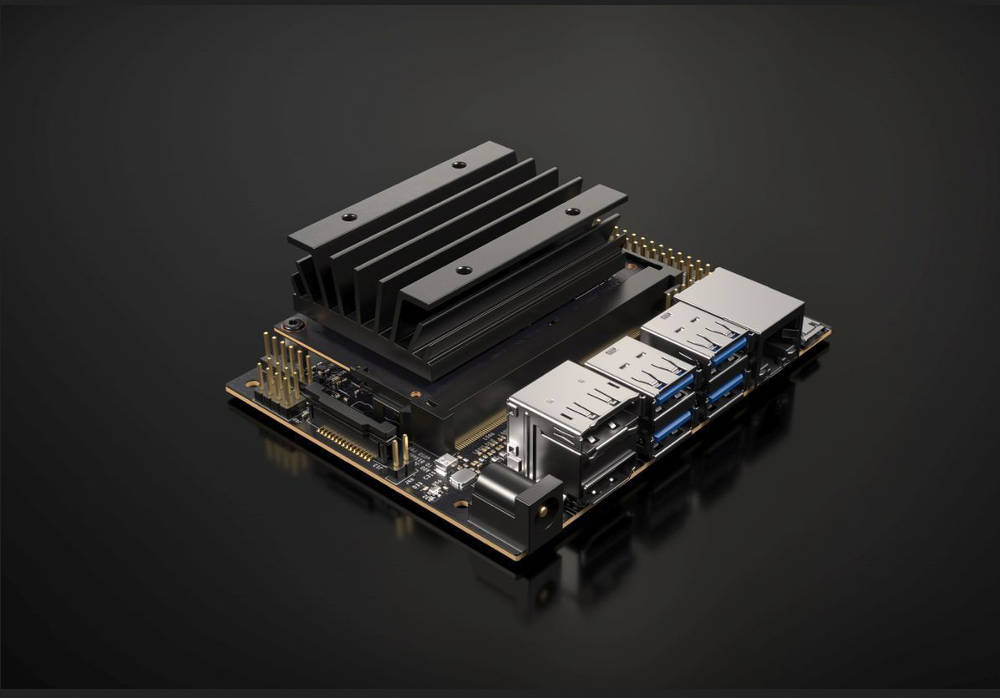# CUDA with Jetson Nano – Parallel Pollard Rho testMath is hard. When your teacher does not like to teach it.

According to Wikipedia,

CUDA (Compute Unified Device Architecture) is a parallel computing platform and application programming interface (API) model created by Nvidia. It allows software developers and software engineers to use a CUDA-enabled graphics processing unit (GPU) for general purpose processing — an approach termed GPGPU (General-Purpose computing on Graphics Processing Units). The CUDA platform is a software layer that gives direct access to the GPU’s virtual instruction set and parallel computational elements, for the execution of compute kernels.

In other, simpler words, Nvidia delivered a new development framework, made of both hardware and software, to parallelize computing tasks, thus reducing their completion times.

The CUDA paradigm is composed of a dedicated hardware with multiple processors, and a clever extension of the C/C++ language, capable to address the power of each and every single processor through simple tasks. When you have to perform repetitive tasks like searches, queries, sorting and convolutions, you can get (partially) rid of heavy loops, and schedule a tiny list of operations to different workers. With thousands of worker threads acting in parallel, the time to complete the task is greatly reduced.Of course there are some caveats: while the serial Von Neumann scheduling only requires an input, a black box where the elaboration is done instruction after instruction, and an output, parallel architectures need to consider where the data is stored, who is working on it (and how), when will some data be free for the next evaluation. The analysis of concurrency is not easy, but once you know the basis it becomes straightforward.

#### CUDA Hardware – The Jetson Nano

CUDA reached its 10th incarnation, showing as a powerful and stable development tool. Born as a graphical architecture able to quickly address pixels and drive their colors on a monitor, CUDA has today become a complete system for parallel development. While its share of fame still resides in graphical data treatment and imaging, an ever growing group of developers dedicated time and effort to expose and exploit the parallel power hidden in the bare metal. Engineers and math researchers refactored well-known serial algorithms to harness the blazing speed of multiprocessing.

And rightly so. We have developed a parallel factorization algorithm witn CUDA GPUs, up to 1,000x faster than the original CPU method. Just dedicating one thread to each one of the 960 residual classes (out of 4620) we have such huge speedup. But the Jetson Nano does not have 960 spare computing units, and although it could still benefit from its 128 CUDA cores, we will test a different factorization algorithm on it.

Instead of subdividing the work into 8 blocks using 125 cu each, we will try a totally different approach, a bit less deterministic, but that will take advantage of the somewhat limited CUDA with Jetson Nano using a statistics simulation.#### Factorization – The Pollard Rho algorithm

From Wikipedia:

Suppose we need to factorize a number n = p*q, where p is a non-trivial factor. A polynomial modulo n, called g(x) (e.g., g(x) = (x2 + 1) mod n, is used to generate a pseudorandom sequence. A starting value, say 2, is chosen, and the sequence continues as x1=g(2), x2=g(g(2)), x3=g(g(g(2))), etc. The sequence is related to another sequence {xk mod p}. Since p is not known beforehand, this sequence cannot be explicitly computed in the algorithm. Yet, in it lies the core idea of the algorithm.

Because the number of possible values for these sequences are finite (the modular operation assures it), both the {xk} sequence, which is mod n, and {xk mod p } sequence will eventually repeat, even though we do not know the latter. Assume that the sequences behave like random numbers. Due to the birthday paradox (we will analyze it o another article), the number of xk before a repetition occurs is expected to be O(√N), where N is the number of possible values. So the sequence {xk mod p} will likely repeat much earlier than the sequence {xk}. Once a sequence has a repeated value, the sequence will cycle, because each value depends only on the one before it. This structure of eventual cycling gives rise to the name “Rho algorithm”, owing to similarity to the shape of the Greek character ρ when the values x1 mod p, x2 mod p, etc. are represented as nodes in a directed graph.

Ok. Now let’s take a deep breath, and see what the previous paragraph really means.

Suppose we are running on a long circular race track. How do we know we have completed one cycle? A clever solution is to have two runners A and B with B running twice as fast as A. They start off at the same position and when B overtakes A, we know that B has cycled around at least once.

We have the following algorithm:

Suppose we want to factor the number 8051. We have

Applying the algorithm, we get the following resolution steps:

iterationx mod 8051y = (x^2 + 1)^2 + 1 mod 8051GCD(|x − y|, 8051)
1221
25 (2^2 +1)26 (5^2 + 1)1
326 (5^2 + 1)7474 ((677^2 + 1) mod 8051)1
4677 (26^2 + 1)87197

Starting with different x and/or y, we could find the other divisor of 8051, 83

If we use a naive trial-factoring algorithm, we should test all prime factors below the square root of N. The trial-factoring algorithm grows exponentially with the digits of the number (2n/2) .

The Pollard Rho algorithm offers a trade-off between its running time and the probability that it finds a factor. A prime divisor can be achieved with a probability around 0.5, in O(√d) <= O(n1/4) iterations. This is a heuristic claim, and rigorous analysis of the algorithm remains open.

#### From theory to practice

To show the algorithm in action (and how a parallel implementation can accelerate outcomes) we prepared a short source code, running the Pollard Rho factorization using one CPU, multiple CPUs through OpenMP, and GPUs. A list of candidates to factorize is provided, to show:

1. How the algorithm becomes slower as the factor grows
2. How the slowdown is due to the factor size, and not to the number to factor
3. How the GPU overhead makes the parallel algorithm slower than the CPU’s for smaller factors
4. How a correct choice of the blocksize and blocknumber for GPUs can lead to better results
5. What is the overall gain of the parallel algorithm.The algorithm we present is limited to 64 bit numbers, but it is fairly easy to extend it to multi-precision arithmetic. Anyway, it is known that the efficiency of Pollard Rho decreases with the length of the factors: in general, when N has more than 40 digits, other methods (such as P-1 and ECM) should be chosen.

The algorithm is very fast for numbers with small factors, but slower in cases where all factors are large. The ρ algorithm’s most remarkable success was the factorization of the eighth Fermat number, F8 = 1238926361552897 * 93461639715357977769163558199606896584051237541638188580280321. The ρ algorithm was a good choice for F8 because the prime factor p = 1238926361552897 is much smaller than the other factor. The factorization took 2 hours on a UNIVAC 1100/42.

You will find more information on Fermat factorization and primality proving on my FermatSearch site.

#### Differences between CPU and GPU implementation

We have two different kernels to run as host and device.

The host kernel runs on the CPU (or CPUs when OpenMP is enabled). It takes the number(s) to be factored, and loops until a solution is found.

The device kernel runs on the GPU. The (random) values of x and y are pre-computed, indexed and stored inside an array. The array is copied asynchronously after the number to factor. Then the block is launched. Each thread of the device code simultaneously accesses a section of the array, calculates its step (g(x), g(g(x)), absolute value of the sum and gcd), then stores the results back on the array, and checks if the GCD is greater than 1. If it is, we have a factor. The value is saved on the result location. Once this one-pass loop is completed, the result is transferred back to the host memory, and returned to the calling main.

#### How does it perform?

We used a list of 19 numbers, and tested them on 2 different platforms: a PC with a Intel Core i7 9800X processor and a Nvidia RTX 2060 GPU, and a Nvidia Jetson Nano board.

The PC gave the following results:

In other words, even a naive implementation of the algorithm in CUDA showed a speedup of 320x. We ran a 256×256 grid on the GPU while we had 1920 computational units, and didn’t take advantage of shared memory speedup or register optimization.

On the Nano we achieved the following timings:

The GPU on the Nano is a Maxwell with a low number of cores and aa clock below 1 GHz, but we achieved a 48x speedup relative to the CPU.

We also notice that CUDA with Jetson Nano using the parallel algorithm is more than 12 times faster than the Intel 9800X. Not bad for a board that costs 15 times less than the PC system.

The source code to experiment CUDA with Jetson Nano is available on Github. We will test other back-to-back CPU vs GPU algorithms soon, to show not only the joy of parallel computing, but also the amaze of the graphic acceleration of CUDA.